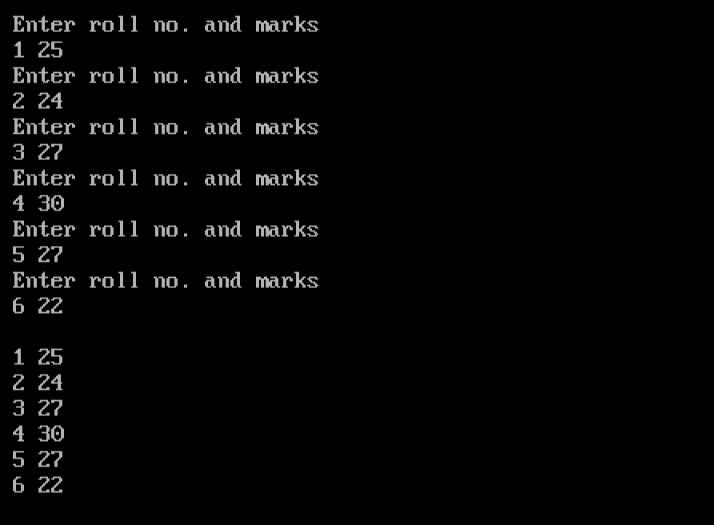# Arrays in C (2D Array) – Part 5

Till now I told you about the 1D arrays but today I will discuss about the 2D arrays or two dimensional arrays in c. Array is a collection of elements with similar data type. We can make arrays with any dimension. However programmers rarely go beyond 3D arrays. I will also give you an overview on 3D arrays in the subsequent tutorials. But today lets discuss the 2D arrays briefly.

## 2D Arrays in C

As its name suggests, 2D arrays are the arrays having 2 dimensions. Those two dimensions are generally called rows and columns. 2D arrays are also called matrix.

### Declaration of 2D Array

A two dimensional array can be declared in following way.

int a;

This is a 2D array with 3 rows and 3 columns. The total elements in the array are 9 (3×3).

### Initialization of 2D Array

Similar to 1D arrays, a 2D array can also be initialize at the time of its declaration. Given below are some of the initialization methods that are used very frequently.

int num = {
{43,56},
{56,54},
{65,98}
};

This is one of the simplest way of initializing a 2D array.

int num = {43, 56, 56, 54, 65, 98};

This method will also work but it will decrease the readability of the array too.

int  arr[ ] = { 12, 34, 23, 45, 56, 45 } ;
int num[] = {
{43,56},
{56,54},
{65,98}
{87,86}
};

It is optional to provide the row dimension of a 2D array if we initialize it. Remember giving column dimension is always compulsory.

Lets take one simple program to understand 2D array in C.

OutputExplanation
1. In the first statement I have declared the 2D array with name student. Remember 2D array also stores elements with index 00. Elements will be stored in this way.

00 01
10 11
20 21
And so on.

2. Now by using two for loops I have stored the values inside 2D array and display it on the screen.

3. Consider carefully the printf() and scanf() function with arguments “&stud[x], &student[x]”. We are storing and accessing values inside 2D array by row wise. We can also store values and access them column wise too.

### Memory Allocation of 2D Array

2D arrays also stores its values similar to 1D arrays. They also store elements in contiguous memory locations.

Elements of first row will be stored first, after that elements of second row will be stored. This procedure will continue until the array elements ends. Given below is the memory allocation of a 2D integer array s.

### You May Also Like:

Category: C Tutorials